﻿ 基于ARMA模型的碳排放权交易价格波动实证分析—以深圳排放权交易为例 The Empirical Study of Carbon Emission Price’s Volatility Based on ARMA Model—Evidence from Shenzhen Emissions Exchange

Statistics and Application
Vol.06 No.04(2017), Article ID:22480,10 pages
10.12677/SA.2017.64048

The Empirical Study of Carbon Emission Price’s Volatility Based on ARMA Model

—Evidence from Shenzhen Emissions Exchange

Peng Cheng, Xuehua Zhang*

Institute of Environmental Economics, Tianjin Polytechnic University, Tianjin

*通讯作者。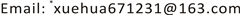Received: Oct. 4th, 2017; accepted: Oct. 20th, 2017; published: Oct. 27th, 2017ABSTRACT

Environmental problems are becoming increasingly serious. It has been agreed by the world that we are supposed to develop the real economy by promoting the development of the low-carbon economy. This paper uses data of the average price of carbon emissions in Shenzhen Carbon Exchange and analyzes carbon emission price’s volatility of return by ARCH models based on ARMA model. The results show that there is a certain degree of time lag and obvious conditional heteroscedasticity effects in carbon emission rate, which could be corrected and precisely described by ARMA-GARCH model. Finally we use the revised model to forecast the market price in recent period.

Keywords:ARMA Model, Carbon Emission, Volatility of Return, ARCH Models

—以深圳排放权交易为例1. 引言

2011年10月，“十二五”规划中关于逐步建立国内碳排放权交易市场的要求，同意北京市、天津市、上海市、重庆市、湖北省、广东省及深圳市开展碳排放权交易试点。2013年6月18日，我国首家碳排放权交易所于深圳成立，并进行碳排放权的交易。2014年，7个试点已经全部启动上线交易，2015年以来，市场规模扩大，覆盖的试点企业比上年增加约400个。

2015年6月17日下午，“第三届深圳国际低碳城论坛”碳交易论坛在深圳国际低碳城召开。本次论坛结合我国“一带一路”战略构想与碳交易作为应对全球气候变化的创新实践，首次提出“绿色一带一路”的观念，以减排温室气体、实现共同低碳发展为目标，利用深圳碳市场的实践经验，以碳交易合作为手段，推动国内碳市场的建设和“一带一路”地区的低碳发展和应对气候变化工作，丰富了国家“一带一路”战略的内涵。

《巴黎协定》于2015年12月12日在气候变化巴黎大会上通过。2016年4月22日，中美同时签署《巴黎协定》。中国作为世界上最大的发展中国家，展现了大国应对全球环境问题的决心。

2. 模型构建

2.1. 模型介绍

2.1.1. 自回归移动平均模型

${Y}_{t}={\varphi }_{1}{Y}_{t-1}+{\varphi }_{2}{Y}_{t-2}+\cdots +{\varphi }_{p}{Y}_{t-p}+{u}_{t}$

${Y}_{t}={u}_{t}+{\theta }_{1}{u}_{t-1}+{\theta }_{2}{u}_{t-2}+\cdots +{\theta }_{q}{u}_{t-q}$

${Y}_{t}={\varphi }_{1}{Y}_{t-1}+{\varphi }_{2}{Y}_{t-2}+\cdots +{\varphi }_{p}{Y}_{t-p}+{u}_{t}+{\theta }_{1}{u}_{t-1}+{\theta }_{\text{2}}{u}_{t-\text{2}}+\cdots +{\theta }_{q}{u}_{t-q}$

2.1.2. ARCH族模型

${y}_{t}=\underset{i=1}{\overset{n}{\sum }}{\beta }_{i}{x}_{it}+{u}_{t}$

${\sigma }_{t}^{2}={\alpha }_{0}+{\alpha }_{1}{u}_{t-1}^{2}+{\alpha }_{2}{u}_{t-2}^{2}+\cdots +{\alpha }_{p}{u}_{t-p}^{2}$

ARCH模型可以解决时间序列中的异方差，但在实际应用中常出现 ${u}_{t}$ 依赖于很多时刻之前的变化量，即拟合模型需要高阶的ARCH，而这很难精确做到。考虑到ARCH模型中的方差方程是 ${\sigma }_{t}^{2}$ 的一个分布滞后模型，就可以用一个或多个 ${\sigma }_{t}^{2}$ 的滞后值代替许多 ${u}_{t}^{2}$ 的滞后值，Bollerslev (1986)提出广义自回归条件异方差模型，即GARCH模型。GARCH(p,q)模型定义如下：

${y}_{t}=\underset{i=1}{\overset{n}{\sum }}{\beta }_{i}{x}_{it}+{u}_{t}$

${\sigma }_{t}^{2}=\omega +\underset{j=1}{\overset{q}{\sum }}{\beta }_{j}{u}_{t-j}^{2}+\underset{i=1}{\overset{p}{\sum }}{\alpha }_{i}{u}_{t-i}^{2}$

2.2. 参数回归

2.2.1. 数据平稳化处理

2015年4月2日至2017年4月19日，我国深圳排放权交易所的碳排放权成交均价序列的均值为35.35155，极大值为49.06000，极小值为17.59000，其标准差为6.634856，成交均价序列(P)时序图见图1，相关图和偏相关图见图2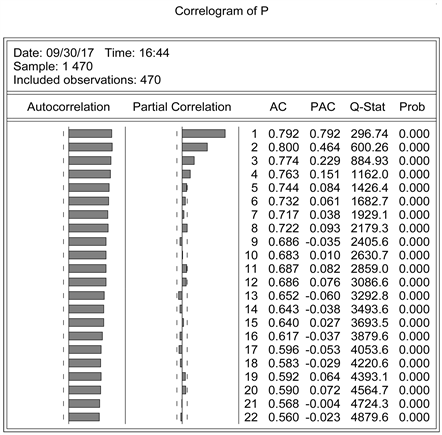Figure 2. Correlogram of P

2.2.2. 特征数求解

2.2.3. 自回归检验

2.2.4. 自回归修正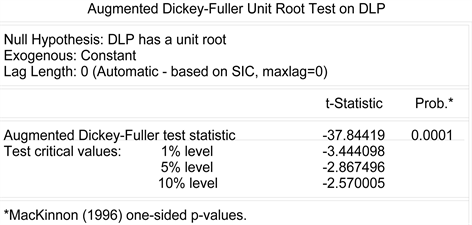Figure 4. Augmented dickey-fuller unit root test on DLP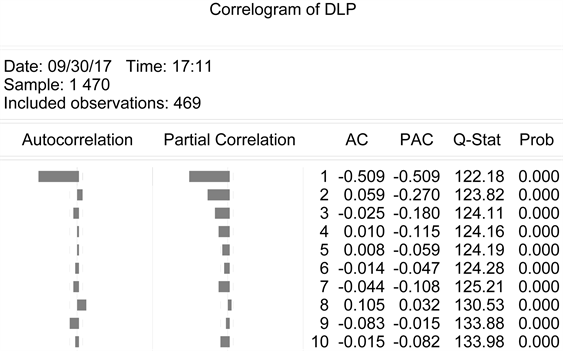Figure 5. Correlogram of DLP

ARMA模型估计对参数t检验显著性水平要求并不严格，更多的是考虑模型的整体拟合效果。根据AIC准则和SC准则，AIC和SC的值都越小越好，因此综合可决系数、AIC和SC准则，本文认为ARMA(02,1)模型是较优选择  。说明碳排放权交易价格收益率与其二阶滞后变量有一定的相关性，且存在较为明显的一阶移动平均过程，这说明碳排放权收益率存在一定的时滞性。

Eviews8.0输出结果为图6

$DL{P}_{t}=0.065DL{P}_{t-2}-0.732{u}_{t-1}+{u}_{t},\text{\hspace{0.17em}}\text{\hspace{0.17em}}\text{\hspace{0.17em}}\text{\hspace{0.17em}}{R}^{2}=0.354064$

2.2.5. 条件异方差检验和修正

$DL{P}_{t}=\epsilon DL{P}_{t-2}+\eta {u}_{t-1}+{u}_{t}$

${\sigma }_{t}^{2}=\omega +{\alpha }_{1}{u}_{t-1}^{2}+{\alpha }_{2}{u}_{t-2}^{2}+\beta {\sigma }_{t-1}^{2}$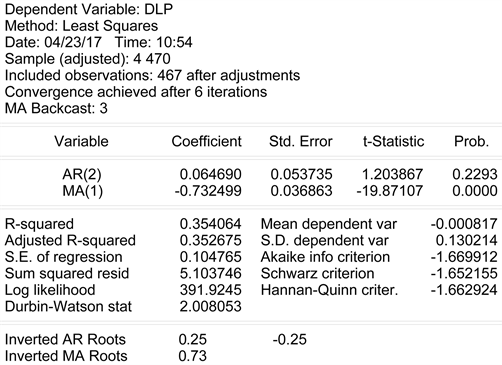Figure 6. Estimation results in model ARMA(02,1)

$DL{P}_{t}=0.067DL{P}_{t-2}-0.680{u}_{t-1}+{u}_{t}$

$\begin{array}{l}{\sigma }_{t}^{2}=0.001+0.420{u}_{t-1}^{2}-0.296{u}_{t-2}^{2}+0.842{\sigma }_{t-1}^{2}\\ \text{\hspace{0.17em}}\text{\hspace{0.17em}}\text{\hspace{0.17em}}\text{\hspace{0.17em}}\text{\hspace{0.17em}}\text{\hspace{0.17em}}\text{\hspace{0.17em}}\left(2.61\right)\text{\hspace{0.17em}}\text{\hspace{0.17em}}\text{\hspace{0.17em}}\text{\hspace{0.17em}}\left(6.74\right)\text{\hspace{0.17em}}\text{\hspace{0.17em}}\text{\hspace{0.17em}}\text{\hspace{0.17em}}\text{\hspace{0.17em}}\text{\hspace{0.17em}}\text{ }\left(-3.50\right)\text{\hspace{0.17em}}\text{\hspace{0.17em}}\text{\hspace{0.17em}}\text{\hspace{0.17em}}\text{\hspace{0.17em}}\text{\hspace{0.17em}}\left(15.74\right)\end{array}$

$DL{P}_{t}=0.068DL{P}_{t-2}-0.680{u}_{t-1}+{u}_{t}-0.008\sqrt{{\sigma }_{t}^{2}},\text{\hspace{0.17em}}\text{\hspace{0.17em}}\text{\hspace{0.17em}}{R}^{2}=0.350134$

GARCH(1,2)的方程为: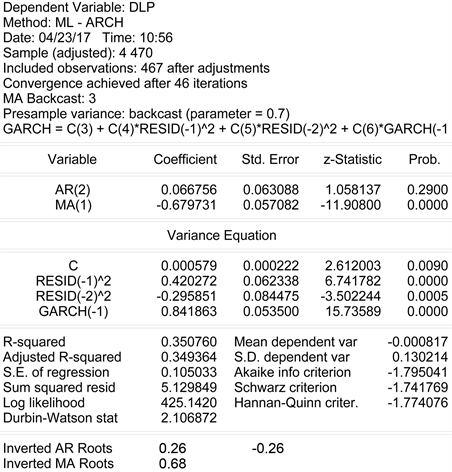Figure 7. Estimation results in model GARCH(1,2)

${\sigma }_{t}^{2}=0.001+0.423{u}_{t-1}^{2}-0.298{u}_{t-2}^{2}+0.839{\sigma }_{t-1}^{2}$

GARCH(1,2)模型拟合结果表明：碳排放权价格收益率波动会受到误差项二阶滞后和波动的一阶滞后的影响，a值表示市场外部环境因素对收益率的影响，b值表示收益率波动对其自身的影响，b值越大表明收益率波动对其自身的影响越长远，即具有长期记忆性  。b = 0.842，说明冲击的84.2%会持续到下期，且影响系数加总求和得到0.966 < 1，说明碳排放权价格收益率序列具有有限方差，而且波动最终会衰减。

3. 碳排放权价格短期预测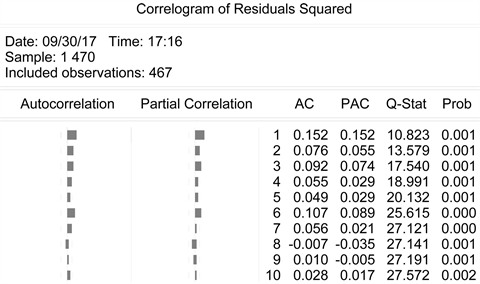Figure 8. Correlogram of residuals squared in model ARMA(02,1)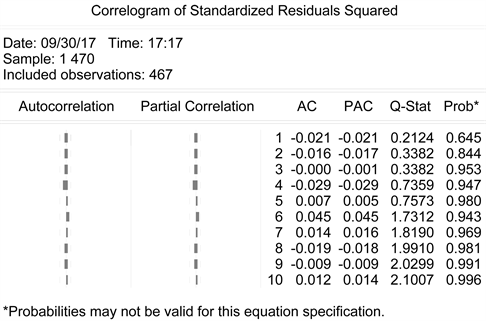Figure 9. Correlogram of residuals squared in model ARMA(02,1)- GARCH(1,2)Table 3. Short term prediction of carbon emission price

4. 结论

1) 碳排放权收益率存在一定的时滞性，而且该时滞性可以被ARMA模型较为准确地描述并进行修正。当期受滞后两期的影响最为显著，这可能是由于当前国内碳排放权交易市场交易制度不够完善，碳排放权的交易现在还没有全面向个人和普通投资者放开，更多情况下只针对能耗大、污染大而且对碳排放权有实际需求的企业。

2) 均值方程和方差方程都显示收益率及其波动性与其二阶滞后项有明显的相关性，这可能和碳排放权市场的不成熟有关，导致当期碳排放权的收益率及其波动性不能及时反映其前一期及其他期的信息。但是这种相关性可以被较为准确地描述从而进行近几期市场价格的预测。

3) 政府应明确自身与市场的边界，以市场化为主导，随着我国碳交易现货市场的不断深入，可以着手建立期货期权市场以完善我国的碳交易市场。政府可以通过合理预测碳交易价格，调控碳市场交易，严格监测碳价波动，建立风险防控体系，完善碳交易相关法律法规，规范碳市场体系。

4) 由于市场不确定因素较多，投资者在投资时容易产生羊群效应，对收益率的波动更为敏感，在波动性大的时候倾向于大幅地调整自身的投资策略，使得碳排放权价格收益率呈现明显的波动集群性特征。投资者也可以通过理性预测碳交易价格，有效规避风险，达到期望收益。

The Empirical Study of Carbon Emission Price’s Volatility Based on ARMA Model—Evidence from Shenzhen Emissions Exchange[J]. 统计学与应用, 2017, 06(04): 418-427. http://dx.doi.org/10.12677/SA.2017.64048

1. 1. 邹亚生, 魏薇. 碳排放核证减排量(CER)现货价格影响因素研究[J]. 金融研究, 2013(10): 142-153.

2. 2. 徐天艳. 基于GARCH 模型的核证减排期货价格波动性研究[J]. 时代金融, 2011(12): 209-211.

3. 3. 付培栋. 碳交易价格波动性实证分析[D]: [硕士学位论文]. 济南: 山东大学, 2014.

4. 4. 周天芸, 许锐翔. 中国碳排放权交易价格的形成及其波动特征——基于深圳碳排放权交易所的数据[J]. 金融发展研究, 2016(1): 16-25.

5. 5. 张晓峒. Eviews 使用指南与案例[M]. 北京: 机械工业出版社, 2007.

6. 6. 邱谦, 郭守前. 我国区域碳金融交易市场的风险研究[J]. 资源开发与市场, 2017(2): 188-193.